Type
Quiz
Book Title
Fundamentals of Corporate Finance Standard Edition 9th Edition
ISBN 13
978-0073382395

### 978-0073382395 Chapter 3 Questions and Problems 16-27

April 3, 2019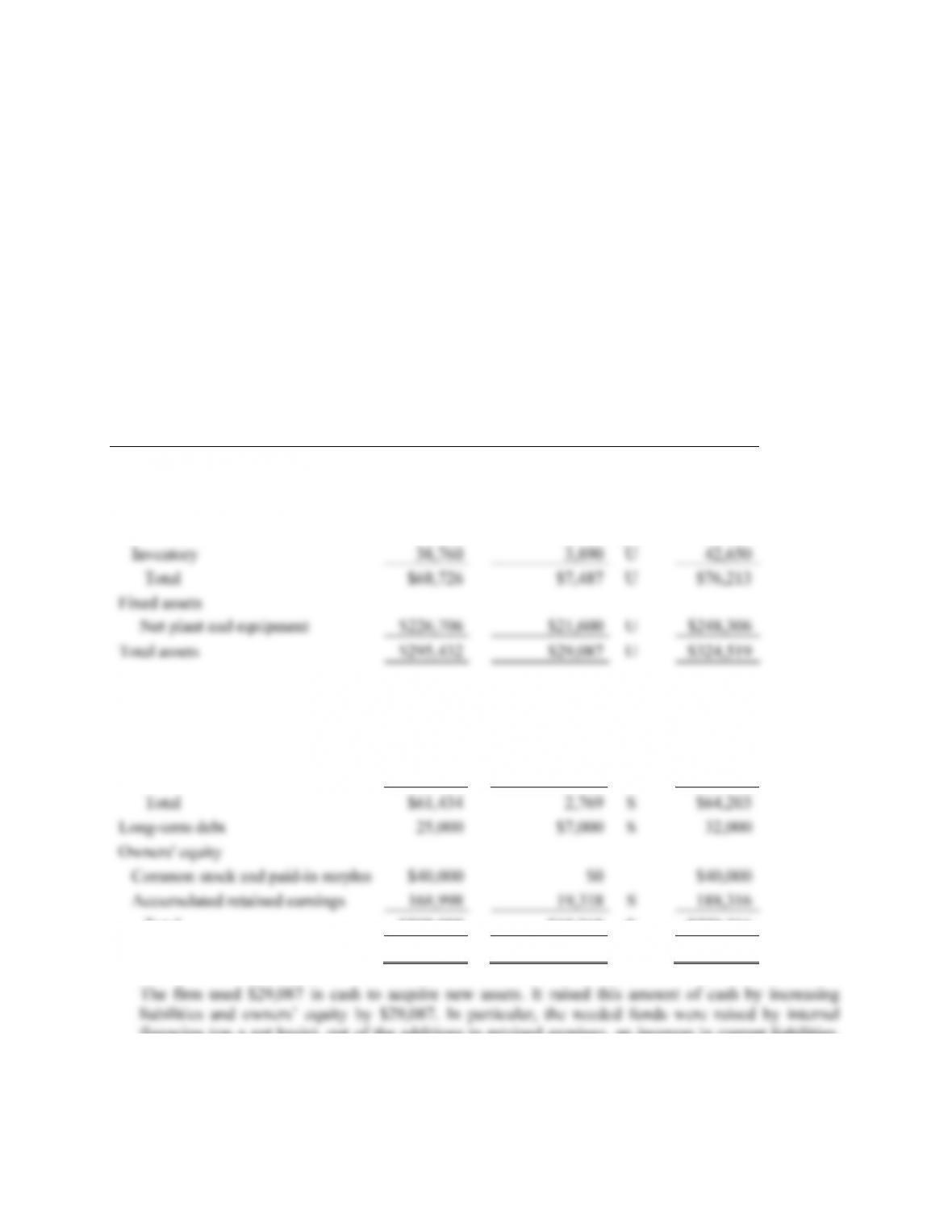CHAPTER 3 B-21
The common-base year answers for Question 14 are found by dividing each category value for 2009
by the same category value for 2008. For example, the cash common-base year number is found by:
\$10,157 / \$8,436 = 1.2040
This means the cash balance in 2009 is 1.2040 times as large as the cash balance in 2008.
The common-size, common-base year answers for Question 15 are found by dividing the common-
size percentage for 2009 by the common-size percentage for 2008. For example, the cash calculation
is found by:
3.13% / 2.86% = 1.0961
This tells us that cash, as a percentage of assets, increased by 9.61%.
16. 2008
Sources/Uses 2008
Assets
Current assets
Cash \$8,436 \$1,721 U \$10,157
Accounts receivable 21,530 1,876 U 23,406
Liabilities and Owners’ Equity
Current liabilities
Accounts payable \$43,050 3,771 S \$46,821
Notes payable 18,384 –1,002 U 17,382
Total \$208,998 \$19,318 S \$228,316
Total liabilities and owners' equity \$295,432 \$29,087 S \$324,519
financing (on a net basis), out of the additions to retained earnings, an increase in current liabilities,
and by an issue of long-term debt.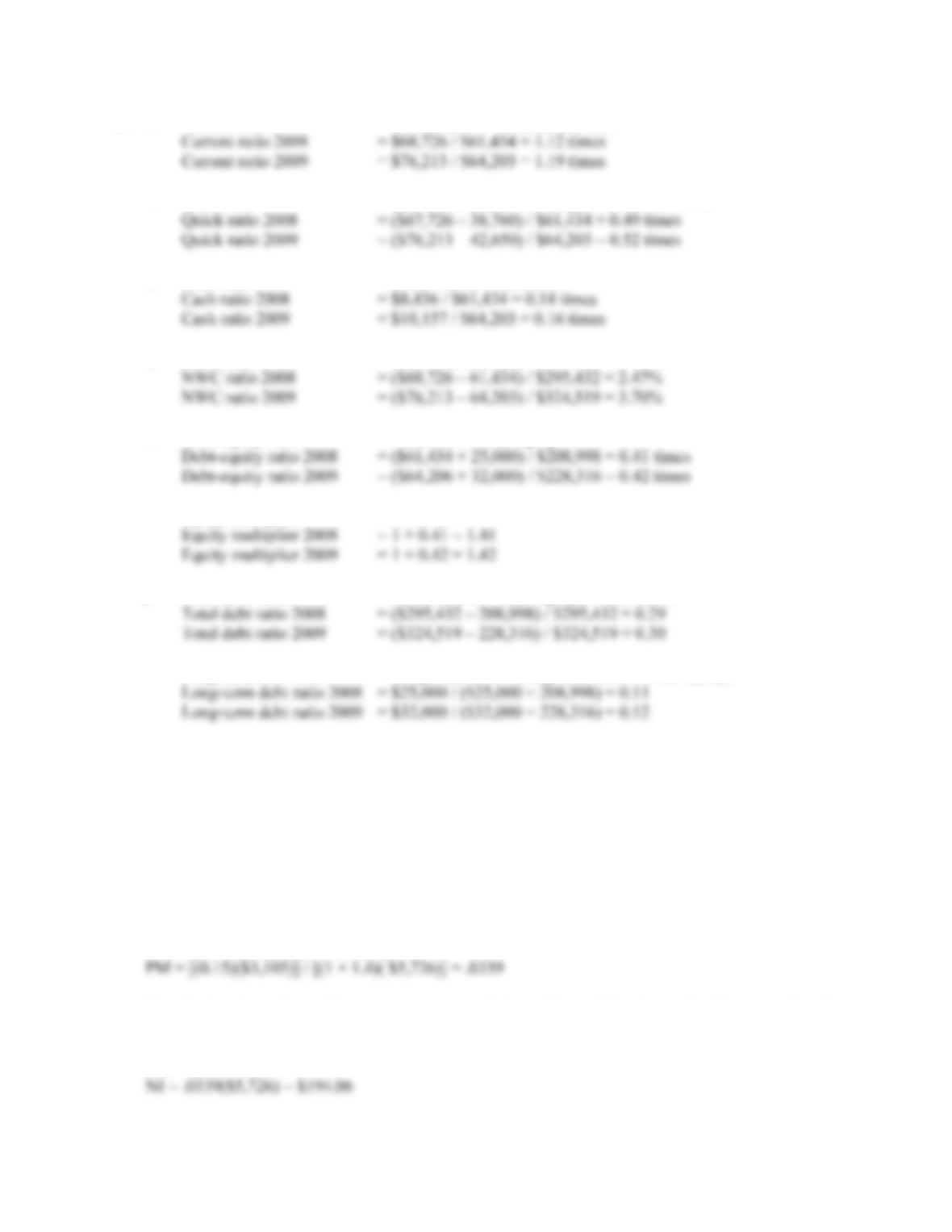B-22 SOLUTIONS
17. a. Current ratio = Current assets / Current liabilities
b. Quick ratio = (Current assets – Inventory) / Current liabilities
c. Cash ratio = Cash / Current liabilities
d. NWC ratio = NWC / Total assets
e. Debt-equity ratio = Total debt / Total equity
Equity multiplier = 1 + D/E
f. Total debt ratio = (Total assets – Total equity) / Total assets
Long-term debt ratio = Long-term debt / (Long-term debt + Total equity)
Intermediate
18. This is a multi-step problem involving several ratios. The ratios given are all part of the DuPont
Identity. The only DuPont Identity ratio not given is the profit margin. If we know the profit margin,
we can find the net income since sales are given. So, we begin with the DuPont Identity:
ROE = 0.15 = (PM)(TAT)(EM) = (PM)(S / TA)(1 + D/E)
Solving the DuPont Identity for profit margin, we get:
PM = [(ROE)(TA)] / [(1 + D/E)(S)]
Now that we have the profit margin, we can use this number and the given sales figure to solve for
net income:
PM = .0339 = NI / S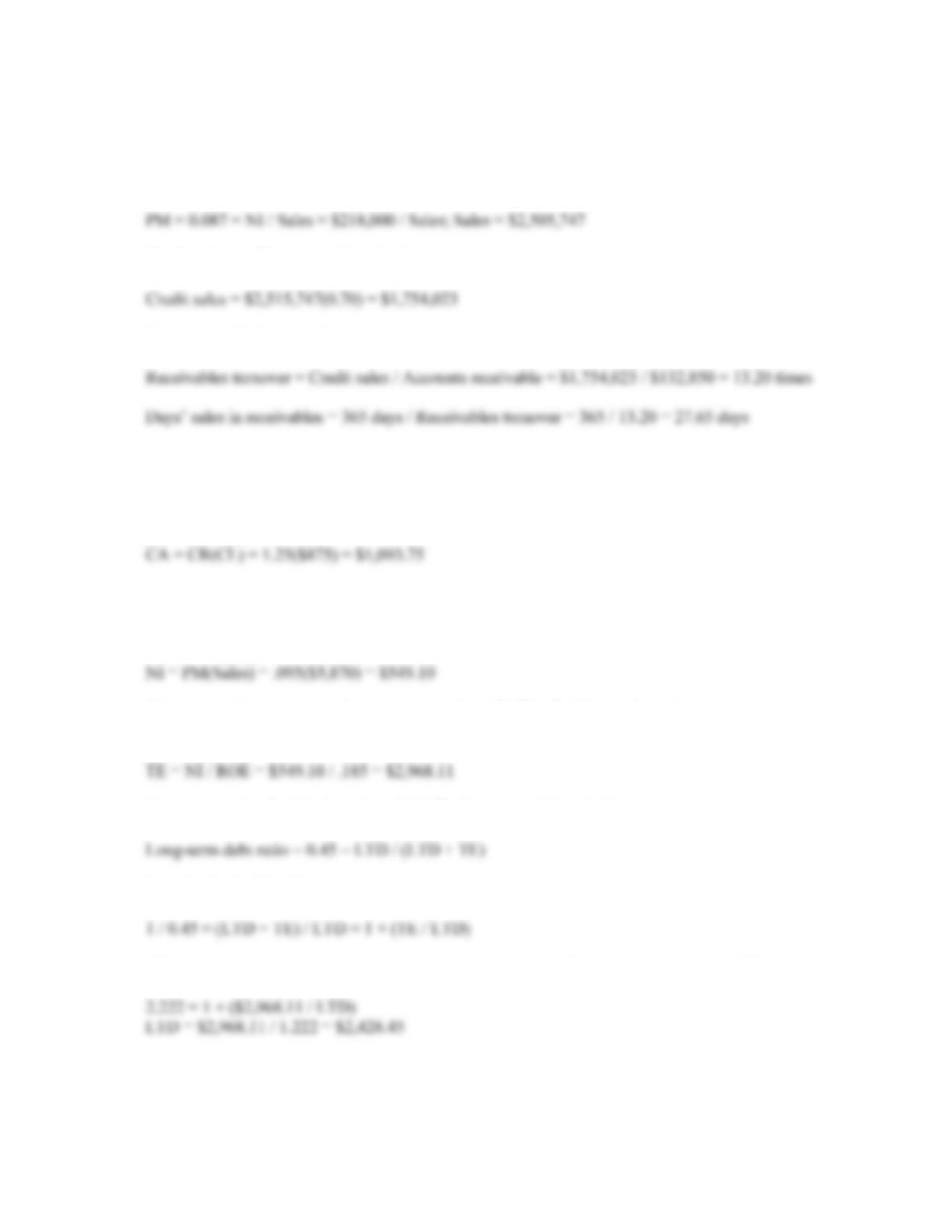CHAPTER 3 B-23
19. This is a multi-step problem involving several ratios. It is often easier to look backward to determine
where to start. We need receivables turnover to find days’ sales in receivables. To calculate
receivables turnover, we need credit sales, and to find credit sales, we need total sales. Since we are
given the profit margin and net income, we can use these to calculate total sales as:
Credit sales are 70 percent of total sales, so:
Now we can find receivables turnover by:
20. The solution to this problem requires a number of steps. First, remember that CA + NFA = TA. So, if
we find the CA and the TA, we can solve for NFA. Using the numbers given for the current ratio and
the current liabilities, we solve for CA:
CR = CA / CL
To find the total assets, we must first find the total debt and equity from the information given. So,
we find the sales using the profit margin:
PM = NI / Sales
We now use the net income figure as an input into ROE to find the total equity:
ROE = NI / TE
Next, we need to find the long-term debt. The long-term debt ratio is:
Inverting both sides gives:
Substituting the total equity into the equation and solving for long-term debt gives the following: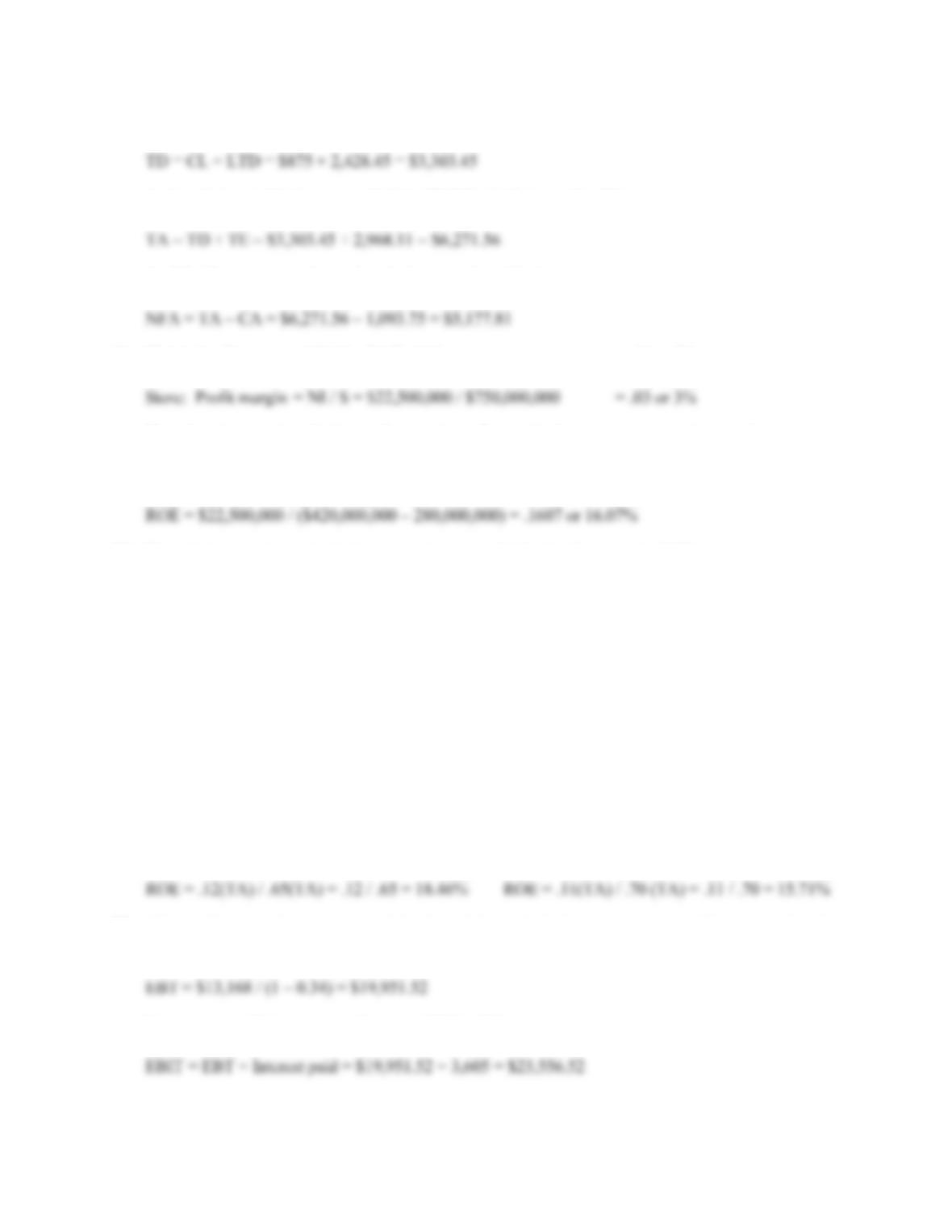B-24 SOLUTIONS
Now, we can find the total debt of the company:
And, with the total debt, we can find the TD&E, which is equal to TA:
And finally, we are ready to solve the balance sheet identity as:
21. Child: Profit margin = NI / S = \$3.00 / \$50 = .06 or 6%
The advertisement is referring to the store’s profit margin, but a more appropriate earnings measure
for the firm’s owners is the return on equity.
ROE = NI / TE = NI / (TA – TD)
22. The solution requires substituting two ratios into a third ratio. Rearranging D/TA:
Firm A Firm B
D / TA = .35 D / TA = .30
(TA – E) / TA = .35 (TA – E) / TA = .30
(TA / TA) – (E / TA) = .35 (TA / TA) – (E / TA) = .30
1 – (E / TA) = .35 1 – (E / TA) = .30
E / TA = .65 E / TA = .30
E = .65(TA) E = .70 (TA)
Rearranging ROA, we find:
NI / TA = .12 NI / TA = .11
NI = .12(TA) NI = .11(TA)
Since ROE = NI / E, we can substitute the above equations into the ROE formula, which yields:
23. This problem requires you to work backward through the income statement. First, recognize that
Net income = (1 – t)EBT. Plugging in the numbers given and solving for EBT, we get:
Now, we can add interest to EBT to get EBIT as follows: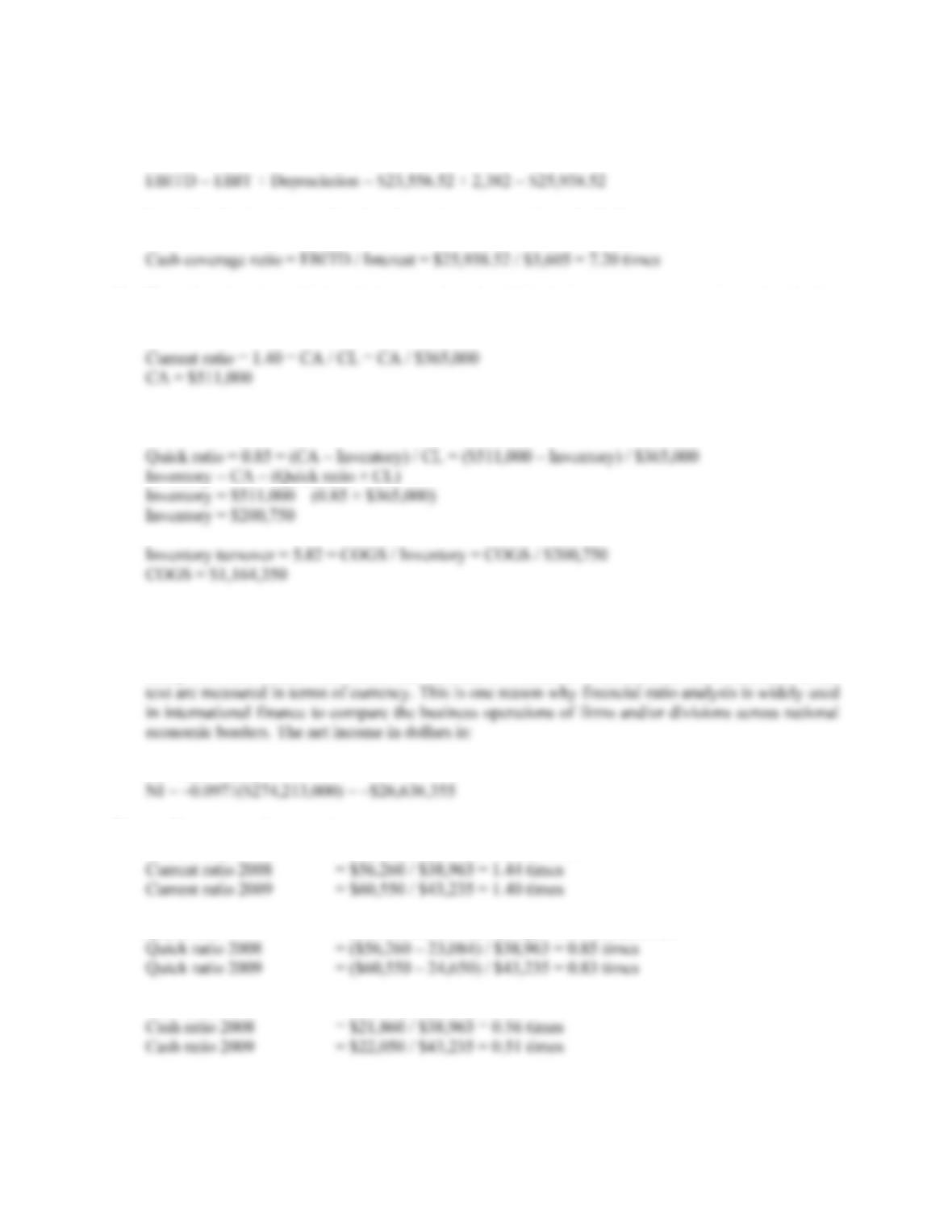CHAPTER 3 B-25
To get EBITD (earnings before interest, taxes, and depreciation), the numerator in the cash coverage
Now, simply plug the numbers into the cash coverage ratio and calculate:
24. The only ratio given which includes cost of goods sold is the inventory turnover ratio, so it is the last
ratio used. Since current liabilities is given, we start with the current ratio:
Using the quick ratio, we solve for inventory:
Inventory = CA – (Quick ratio × CL)
25. PM = NI / S = –£13,482,000 / £138,793 = –0.0971 or –9.71%
As long as both net income and sales are measured in the same currency, there is no problem; in fact,
except for some market value ratios like EPS and BVPS, none of the financial ratios discussed in the
NI = PM × Sales
26. Short-term solvency ratios:
Current ratio = Current assets / Current liabilities
Quick ratio = (Current assets – Inventory) / Current liabilities
Cash ratio = Cash / Current liabilities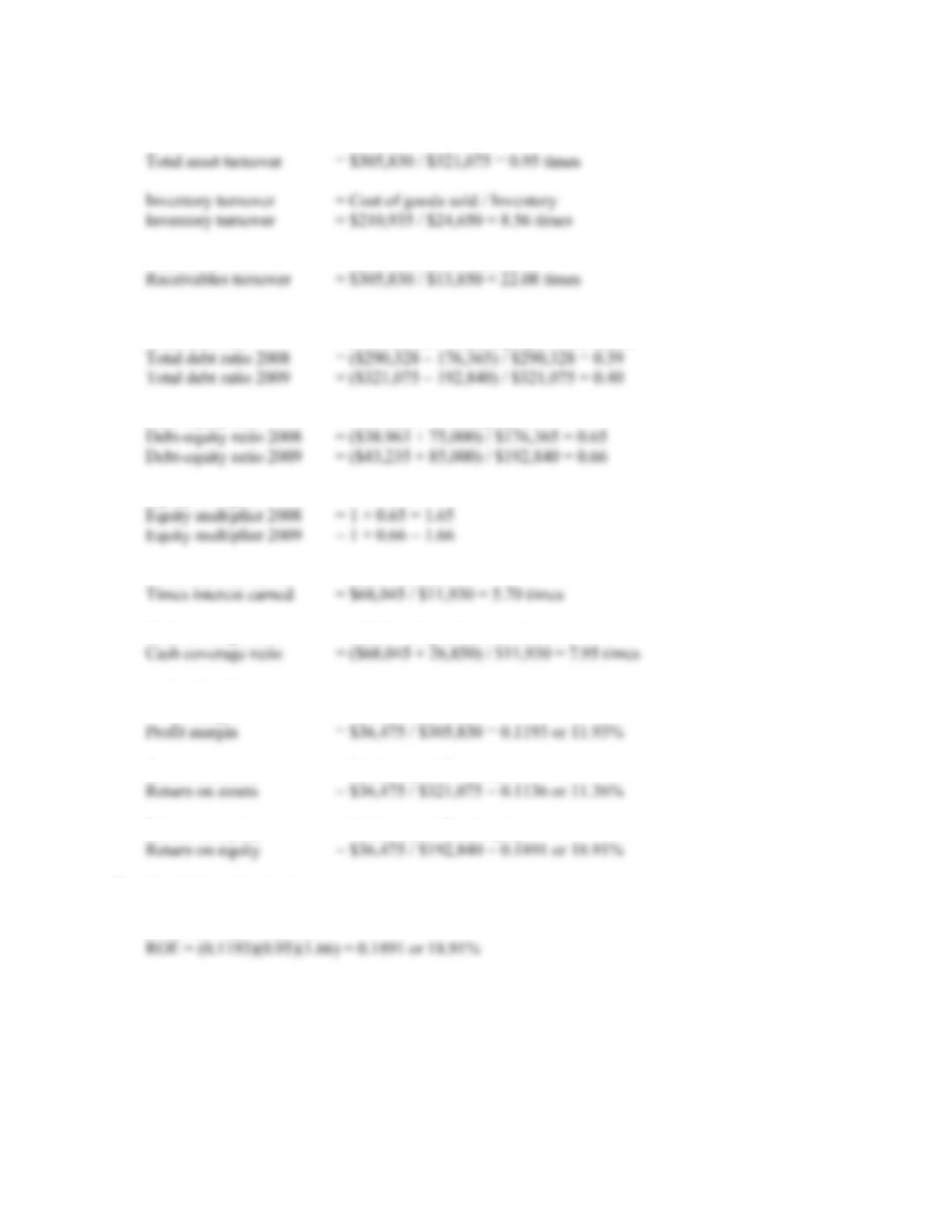B-26 SOLUTIONS
Asset utilization ratios:
Total asset turnover = Sales / Total assets
Receivables turnover = Sales / Accounts receivable
Long-term solvency ratios:
Total debt ratio = (Total assets – Total equity) / Total assets
Debt-equity ratio = Total debt / Total equity
Equity multiplier = 1 + D/E
Times interest earned = EBIT / Interest
Cash coverage ratio = (EBIT + Depreciation) / Interest
Profitability ratios:
Profit margin = Net income / Sales
Return on assets = Net income / Total assets
Return on equity = Net income / Total equity
27. The DuPont identity is:
ROE = (PM)(TAT)(EM)